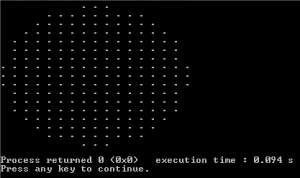Draw a circle without floating point arithmetic

• Difficulty Level : Hard
• Last Updated : 22 Jul, 2021

Given a radius of a circle, draw the circle without using floating point arithmetic.
Following program uses a simple concept. Let the radius of the circle be r. Consider a square of size (2r+1)*(2r+1) around the circle to be drawn. Now walk through every point inside the square. For every every point (x,y), if (x, y) lies inside the circle (or x^2+ y^2 < r^2), then print it, otherwise print space.

C++

 // C++ code to demonstrate to draw// circle without floating// point arithmetic#include  void drawCircle(int r){    // Consider a rectangle of size N*N    int N = 2*r+1;     int x, y;  // Coordinates inside the rectangle     // Draw a square of size N*N.    for (int i = 0; i < N; i++)    {        for (int j = 0; j < N; j++)        {            // Start from the left most corner point            x = i-r;            y = j-r;             // If this point is inside the circle, print it            if (x*x + y*y <= r*r+1 )                printf(".");            else // If outside the circle, print space                printf(" ");            printf(" ");        }        printf("\n");    }} // Driver Program to test above functionint  main(){    drawCircle(8);    return 0;}

Java

 // Java code to demonstrate to draw// circle without floating// point arithmetic class GFG{static void drawCircle(int r){    // Consider a rectangle of size N*N    int N = 2*r+1;     int x, y; // Coordinates inside the rectangle     // Draw a square of size N*N.    for (int i = 0; i < N; i++)    {        for (int j = 0; j < N; j++)        {            // Start from the left most corner point            x = i-r;            y = j-r;             // If this point is inside the circle, print it            if (x*x + y*y <= r*r+1 )                System.out.print(".");            else                // If outside the circle, print space                System.out.print(" ");             System.out.print(" ");        }         System.out.println();    }} // Driver Program to test above functionpublic static void main(String arg[]){    drawCircle(8);}} // This code is contributed// by Anant Agarwal.

Python3

 # Python3 code to demonstrate to draw# circle without floating# point arithmetic def drawCircle(r):     # Consider a rectangle of size N*N    N = 2 * r + 1     # Draw a square of size N*N.    for i in range(N):        for j in range(N):             # Start from the left most corner point            x = i - r            y = j - r             # If this point is inside the circle,            # print it            if x * x + y * y <= r * r + 1:                print(".", end = " ")                             # If outside the circle, print space            else:                print(" ", end = " ")        print() # Driver Codeif __name__ == "__main__":    drawCircle(8) # This code is contributed# by vibhu4agarwal

C#

 // C# code to demonstrate to draw// circle without floating// point arithmeticusing System; public class GFG{    static void drawCircle(int r){    // Consider a rectangle of size N*N    int N = 2*r+1;     int x, y; // Coordinates inside the rectangle     // Draw a square of size N*N.    for (int i = 0; i < N; i++)    {        for (int j = 0; j < N; j++)        {            // Start from the left most corner point            x = i-r;            y = j-r;             // If this point is inside the circle, print it            if (x*x + y*y <= r*r+1 )                Console.Write(".");            else                // If outside the circle, print space                Console.Write(" ");             Console.Write(" ");        }         Console.WriteLine();    }} // Driver Program to test above function    static public void Main (){        drawCircle(8);               }// This code is contributed// by ajit.}



Javascript



Output: